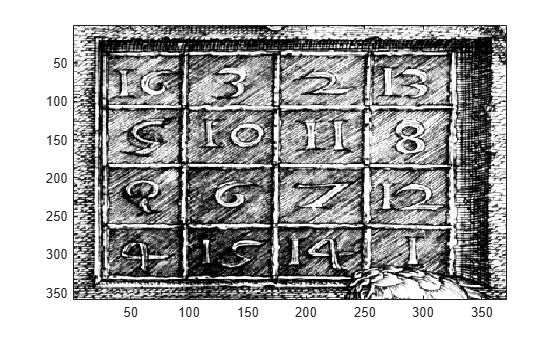# wenergy2

Energy for 2-D wavelet decomposition

## Syntax

``[Ea,Eh,Ev,Ed] = wenergy2(C,S)``
``[Ea,EDetail] = wenergy2(C,S)``

## Description

example

````[Ea,Eh,Ev,Ed] = wenergy2(C,S)` returns, for the 2-D wavelet decomposition structure `C`, `S`: `Ea` — Percentage of energy corresponding to the approximation.`Eh`, `Ev`, and `Ed` — Vectors which contain the percentages of energy corresponding to the horizontal, vertical, and diagonal details, respectively. ```
````[Ea,EDetail] = wenergy2(C,S)` returns `EDetail`, the sum of the energies corresponding to the horizontal, vertical, and diagonal details.```

## Examples

collapse all

```load detail image(X) colormap(map)```Obtain the level 4 wavelet decomposition of the image using the `sym4` wavelet.

```load detail [C,S] = wavedec2(X,4,"sym4");```

Obtain the percentages of energy in the approximation, and all detail levels and orientations.

`[Ea,Eh,Ev,Ed] = wenergy2(C,S)`
```Ea = 86.9903 ```
```Eh = 1×4 1.1921 1.7396 1.8062 1.0492 ```
```Ev = 1×4 1.0084 1.6559 1.5394 1.0467 ```
```Ed = 1×4 0.4793 0.6951 0.5449 0.2528 ```

Confirm the percentage of energy of the combined details equals the sum of the percentages of the individual detail orientations.

`[~,EDetail] = wenergy2(C,S)`
```EDetail = 1×4 2.6799 4.0906 3.8904 2.3488 ```
`sum([Eh;Ev;Ed])`
```ans = 1×4 2.6799 4.0906 3.8904 2.3488 ```

## Input Arguments

collapse all

Wavelet decomposition vector, specified as a vector. `C` contains the approximation and detail coefficients organized by level. The bookkeeping matrix `S` is used to parse `C`. For more information, see `wavedec2`.

Example: `[C,S] = wavedec2(X,3,"db4")` returns the level 4 wavelet decomposition of `X` using the `db4` wavelet.

Data Types: `double`

Bookkeeping matrix, specified as an integer-valued matrix. The matrix `S` contains the dimensions of the wavelet coefficients by level and is used to parse the wavelet decomposition vector `C`. For more information, see `wavedec2`.

Data Types: `double`

## Output Arguments

collapse all

Percentage of energy corresponding to the approximation, returned as a scalar.

Data Types: `double`

Percentage of energy corresponding to the horizontal, vertical, and diagonal details, respectively, returned as 1-by-L vectors, where L is the level of the wavelet decomposition. The kth element of the vector is the percentage of energy at the kth level.

Data Types: `double`

Percentage of energy corresponding to the details, respectively, returned as a 1-by-L vector, where L is the level of the wavelet decomposition. The kth element of the vector is the percentage of energy at the kth level. `EDetail` is the sum of the vectors `Eh`, `Ev`, and `Ed`.

Data Types: `double`

## Version History

Introduced before R2006a